Next: Picturing the Correspondences Up: Lie Algebras Previous: The Adjoint Representation

#### Unitary Matrices: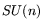Now for a different example.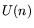is the group of unitary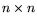matrices, i.e., complex matrices satifying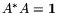. An easy computation shows that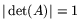.is the subgroup for which the determinant is 1 (unimodular matrices). Unlike the situation with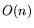and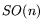, the dimensions ofand(as manifolds) differ by 1.

The Lie algebras ofandare denoted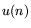and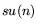, respectively. Differentiatingwe conclude thatconsists of anti-Hermitian matrices: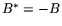. Note that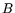is anti-Hermitian if and only ifis Hermitian.

Fact: if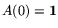, then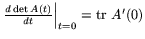(where tr = trace). (Expanding by minors does the trick.) This makes one half of the following fact obvious: the Lie algebra for the Lie group of unimodular matrices consists of all the traceless matrices.

For the special case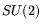, things work out very nicely. Since, one can write down the components foreasily, and equating them to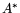, one concludes thatconsists of all matrices of the form: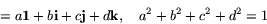defining i,j,kas the givenmatrices in. Exercise: these four elements satisfy the multiplication table of the quaternions, sois isomorphic to the group of quaternions of norm 1. (The somewhat peculiar arrangement of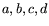in the displayed element ofis dictated by convention.)

Next, an arbitary anti-Hermitian matrix looks like: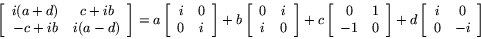This is traceless if and only if. So we have a canonical 1-1 correspondence between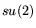and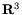, and so also with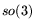: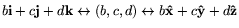.

It turns out that this correspondence is a Lie algebra isomorphism.and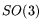are locally isomorphic, but not isomorphic-- as we will see next.acts onvia the adjoint representation. But we have a 1-1 correspondence betweenand, so we have a representation ofin the group of real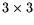matrices. Let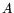be an element ofand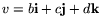be an element of. Note that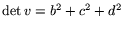. Since the map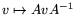preserves determinants, it preserves norms when considered as acting on. So the adjoint representation mapsinto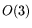. Fact: it mapsonto.

Incidentally, you can see directly thatpreserves anti-Hermiticity by writing it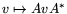.

The kernel of the adjoint representation foris its center, which clearly contains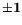-- and in fact, consists of just those two elements. So we have a 2-1 mapping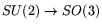. Our double cover! I'll look at the topology of this in a moment.

Physicists prefer to work with the Pauli spin matrices instead of the quaternions. The Pauli matrices are just the Hermitian counterparts to i, j, and k: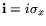,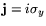,They form a basis (with 1) for the vector space of Hermitianmatrices: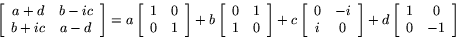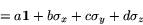acts on the space of traceless Hermitianmatrices in the same way as on: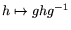.Next: Picturing the Correspondences Up: Lie Algebras Previous: The Adjoint Representation